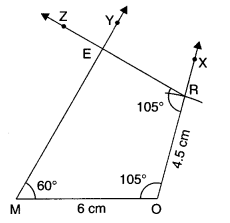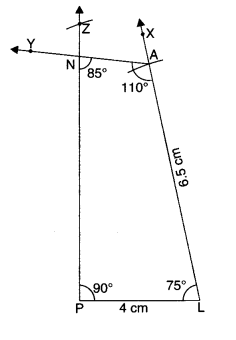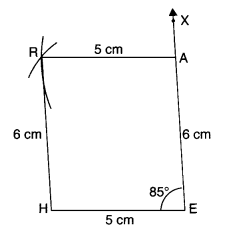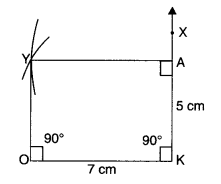NCERT Solutions for Class 8 Maths Chapter 4 Practical Geometry Ex 4.3 are part of NCERT Solutions for Class 8 Maths. Here we have given NCERT Solutions for Class 8 Maths Chapter 4 Practical Geometry Ex 4.3.

 Board CBSE Textbook NCERT Class Class 8 Subject Maths Chapter Chapter 4 Chapter Name Practical Geometry Exercise Ex 4.3 Number of Questions Solved 1 Category NCERT Solutions

## NCERT Solutions for Class 8 Maths Chapter 4 Practical Geometry Ex 4.3

Question 1.
MO = 6 cm
OR = 4.5 cm
∠M = 60°
∠O = 105°
∠R = 105°

PL = 4 cm
LA = 6.5 cm
∠P = 90°
∠A = 110°
∠N – 85°.

(iii) Parallelogram HEAR
HE = 5 cm
EA = 6 cm
∠R = 85°

(iv) Rectangle OKAY
OK = 7 cm
KA = 5 cm.
Solution.
(i) Steps of Construction

1. Draw MO = 6 cm.
2. At 0, draw ray OX such that Z MOX = 105°.3. Cut OR = 4.5 cm from ray OX.
4. At M, draw ray MY such that ∠OMY = 60°.
5. At R, draw ray RZ such that ∠ORZ = 105°.
6. Let the rays MY and RZ meet at E.

Then, MORE is the required quadrilateral.

(ii) Steps of Construction

1. Draw PL = 4 cm.
2. At L, draw ray LX such that ∠PLX = 75°.By Angle-sum property of a quadrilateral,
∠P + ∠A + ∠N + ∠L = 360°
⇒ 90° + 110° + 85° + Z L = 360°
⇒ 285° + ∠ L = 360°
⇒ ∠L = 360° – 285°
⇒ ∠L = 75°.
3. Cut LA = 6.5 cm from ray LX.
4. At A, draw ray AY such that ∠LAY = 110°.
5. At P, draw ray PZ such that ∠LPZ = 90°.
Let the rays AY and PZ meet at N.

Then, PLAN is the required quadrilateral.

(iii) Steps of Construction

1. Draw HE = 5 cm.
2. At E, draw ray EX such that ∠HEX = 85°
∴ Opposite angles of a parallelogram are equal.
∵ ∠E = ∠R = 85°
3. Cut EA = 6 cm from the ray EX.4. With A as centre and radius AR = 5 cm, draw an arc.
5. With H as centre and radius HR = 6 cm; draw another arc to intersect the arc drawn in step 4 at R.
∴ opposite sides of a parallelogram are equal in length
∵ AR = EH = 5 cm
and HR = EA = 6 cm
6. Join AR and HR.

Then, HEAR is the required parallelogram.

(iv) Steps of Construction
[We know that each angle of a rectangle
is 90°.
∴ ∠O=∠K=∠A=∠Y= 90°.
Also, opposite sides of a rectangle are equal in length.
∴ OY = KA = 5 cm and AY = KO = 7 cm]

1. Draw OK = 7 cm.
2. At K, draw ray KX such that ∠OKX = 90°.
3. Cut KA – 5 cm from ray KX.4. Taking A as centre and radius AY = 7 cm, draw an arc.
5. Taking O as centre and radius OY = 5 cm, draw another arc to intersect the arc drawn in step 4 at Y.
6. Join AY and OY.

Then OKAY is the required rectangle.

We hope the NCERT Solutions for Class 8 Maths Chapter 4 Practical Geometry Ex 4.3 help you. If you have any query regarding NCERT Solutions for Class 8 Maths Chapter 4 Practical Geometry Ex 4.3, drop a comment below and we will get back to you at the earliest.